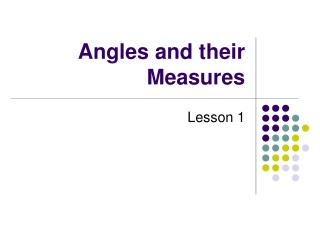DownloadDownload PresentationAngles and their Measures

# Angles and their Measures

Télécharger la présentation## Angles and their Measures

- - - - - - - - - - - - - - - - - - - - - - - - - - - E N D - - - - - - - - - - - - - - - - - - - - - - - - - - -
##### Presentation Transcript

1. Angles and their Measures Lesson 1

2. As derived from the Greek Language, the word trigonometrymeans “measurement of triangles.” • Initially, trigonometry dealt with relationships among the sides and angles of triangles and was used in the development of astronomy, navigation, and surveying.

3. With the development of Calculus and the physical sciences in the 17th Century, a different perspective arose – one that viewed the classic trigonometric relationships as functions with the set of real numbers as their domain. • Consequently the applications expanded to include physical phenomena involving rotations and vibrations, including sound waves, light rays, planetary orbits, vibrating strings, pendulums, and orbits of atomic particles.

4. We will explore both perspectives beginning with angles and their measures….. • An angle is determined by rotating a ray about its endpoint. • The starting position of called the initial side. The ending position is called the terminal side.

5. Standard PositionVertex is at the origin, and the initial side is on the x-axis. Terminal Side Initial Side

6. Positive Angles are generated by counterclockwise rotation. • Negative Angles are generated by clockwise rotation. • Let’s take a look at how negative angles are labeled on the coordinate graph.

7. Negative AnglesGo in a Clockwise rotation

8. Coterminal Angles • Angles that have the same initial and terminal side. See the examples below.

9. Coterminal AnglesThey have the same initial and terminal sides. Determine 2 coterminal angles, one positive and one negative for a 60 degree angle. 60 + 360 = 420 degrees 60 – 360 = -300 degrees

10. Give 2 coterminal angles. 30 + 360 = 390 degrees 30 – 360 = -330 degrees

11. Give a coterminal angle, one positive and one negative. 230 + 360 = 590 degrees 230 – 360 = -130 degrees

12. Give a coterminal angle, one positive and one negative. -20 + 360 = 340 degrees -20 – 360 = -380 degrees

13. Give a coterminal angle, one positive and one negative. Good but not best answer. 460 + 360 = 820 degrees 460 – 360 = 100 degrees 100 – 360 = -260 degrees

14. Complementary AnglesSum of the angles is 90 Find the complement of each angles: 40 + x = 90 x = 50 degrees No Complement!

15. Supplementary AnglesSum of the angles is 180 Find the supplement of each angles: 40 + x = 180 x = 140 degrees 120 + x = 180 x = 60 degrees

16. Coterminal Angles: To find a Complementary Angle:To find a Supplementary Angle:

17. Radian Measure • One radian is the measure of the central angle, , that intercepts an arc, s, that is equal in length to the radius r of the circle. • So…1 revolution is equal to 2π radians

18. Let’s take a look at them placed on the unit circle.

21. More Common Angles Let’s take a look at more common angles that are found in the unit circle.

25. Determine the Quadrant of the terminal side of each given angle. Q1 Go a little more than one quadrant – negative. Q3 A little more than one revolution. Q1

26. Determine the Quadrant of the terminal side of each given angle. Q3 Q2 2 Rev + 280 degrees. Q4

28. Find a coterminal angle. There are an infinite number of coterminal angles!

29. Give a coterminal angle, one positive and one negative.

30. Give a coterminal angle, one positive and one negative.

31. Find the complement of each angles:

32. Find the supplement of each angles:

33. Find the complement & supplement of each angles, if possible: None

34. Coterminal Angles: To find a Complementary Angle:To find a Supplementary Angle: RECAP

35. Conversions